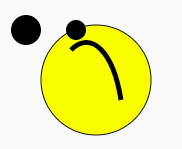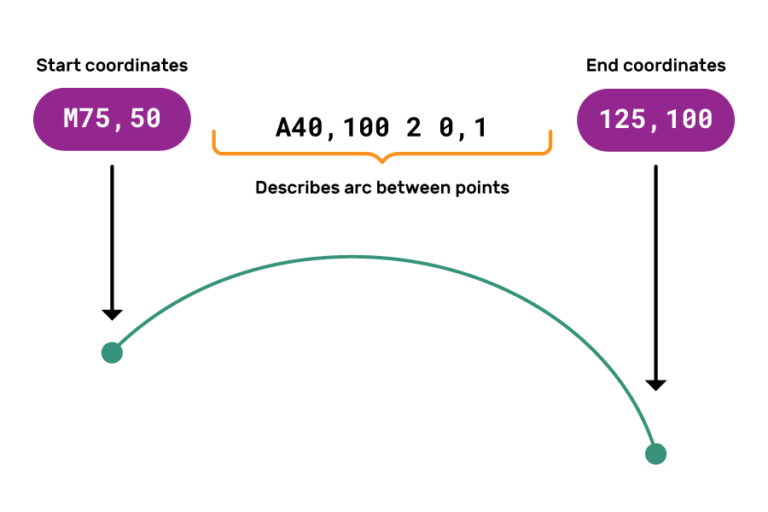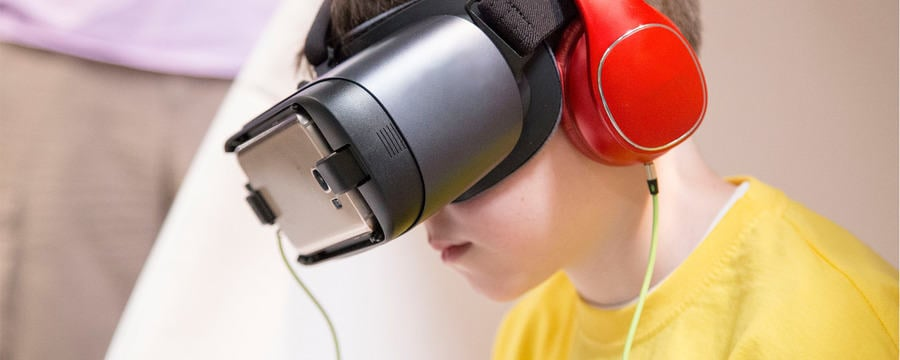£199.99 £139.99 for one year of Unlimited learning. Offer ends on 28 February 2023 at 23:59 (UTC). T&Cs apply

•# Emojis, Bitmap and Vector

Watch Martin O'Hanlon explain how to create your own bitmap and vector emojis using Python code.
2.3
Hi. I’m Martin an educator with the Raspberry Pi Foundation. In this step, I’m going to show you how to create emojis using code. First, let’s have a go at using Python to make a bitmap emoji. Now, let’s start by creating a new Python file in Mu, or by creating a new file Repl.it. I’m going to save my file as Emoji.
28.6
Now, for this exercise, we’re going to be using a Python module called Pillow or Pill. Now, it’s available on Repl.it or pre-installed on Mu. If you’re using a different programming environment, then you may need to install it yourself. Start by importing the modules that you need. We’re going to say from PIL import image.
55.2
Now you need to define some colours. Here I’m just going to create two colours, black and yellow, using the variables b and y. Now, you can define more colours if you would like to. I’ll create my black variable, and I’m going to create my yellow variable.
81.6
Now the creative part. It’s time for you to create your emoji. So I’m going to copy and paste some code I made earlier, which is a list that represents a face. Each letter in the list is the colour of the pixel in the image, either black or yellow. Now, my code will create a smiley face, but you can make whatever emoji you would like. Now, the next bit of code creates a new blank image that is 10 pixels by 10 pixels. This is the same width and height as the face data structure. Now, we can also store that width and height as variables. I’m going to call my image Smiley. I’ll say Smiley equals Image.new. It’s an RGB image.
135.5
And I’m going to make it 10 pixels by 10 pixels. I’m also going to store the width and the height from the smiley’s size.
148.8
Then you need to iterate over the rows and columns of the pixels in the new image. For each pixel, we can change the corresponding RGB value to be the same as those encoded in the face. Now, to do this, I’m going to use two for loops, one inside the other, or nested. I’m going to say for row in range height. And I’ll say for column in range width. And then I’m going to use my smiley image, and I’m going to say put pixel, which is going to go into the column and the row. And I’m going to take the colour from the face list that I created earlier.
202.7
Now, don’t worry if you’re not very familiar with Python and the code is confusing. The important point is that each letter represents a single picture in the final image. To see your image, you can use smiley.save and give your image a name. Again, I’m going to call mine Smiley.jpg. Yep. And also, if you’re on Mu, you can use smiley.show to display your image. Right. Now let’s run our program and see what happens. So we click on Run, and we can see our image. Now, it’s probably a little bit too small to see properly. But don’t forget that we set the image size to be 10 pixels by 10 pixels. Now, we can resize that using this line of code.
258.2
We can say smiley equals smiley.resize and give it a new value. I’m going to make mine 500 pixels by 500 pixels. Now let’s run it again and see what happens.
276.4
I see a much bigger image. Now that you’ve made your first emoji, why not see what other images you can create? Can you add more colours or make the image even larger? Now let’s have a go at creating a vector emoji in HTML. One of the main things to focus on in comparisons in the bitmap emoji is how paths or coordinates are used. When creating emoji features like the mouth, it becomes far more obvious that vectors use coordinates to plot the points. In the article text below, you’ll find a link to the starter Trinket that you’ll need for this project. When you open it up, you’ll see that the face doesn’t look quite right.
316.1
While correcting the face, you’re going to learn how vector paths work. Now, this section of code defines the eyes. r defines the radius of the circle representing each eye, whereas cx and cy define the centre coordinates for each eye. Now, you can change this code to put the eyes in the correct place with the correct size, such as changing 80 to 120. Now, this section of code defines the mouth.
353.9
The d attribute is used to create the vector path within which each element will either be an instruction or a coordinate. Now, if we imagine using a pen to draw the path, the m command says pick up the pen and move it to these positions on the page. By changing these coordinates– M75, comma, 50– you can change where the drawing starts. Now, to change the shape of the mouth, we alter these values.
385.5
And finally, 125, comma, 100 controls the n coordinates of the path. Now play with these coordinates and see what happens. Perhaps share your emoji creations in the comments below. You can also discuss how you might adapt these activities for the classroom to make them easier or extend them or make them more difficult.

You will now put what we discussed in the last step to use by creating some emoji. This will be a hands-on activity that you can use in the classroom to demonstrate some of the theory we have just covered.

We can create images as either bitmap or vector images. To see how this works, you will:

• Create a bitmap emoji using Python
• Create a vector emoji using HTML

## Activity: make a bitmap emoji

• Create a new Python program in repl.it or your IDE.
• Start by importing the PIL module from the pillow package:
from PIL import Image


Note – see the previous step if you haven’t installed pillow.

• Next, define some colours. I’m defining the colours black and yellow using the variables b and y. You can define more colours if you want to.

from PIL import Image

b = (0,0,0)
y = (255,255,0)


• Now it’s time to create your emoji using a list. I’m creating a smiley face, but you can make your own design if you like. Each letter in the list represents the colour of the pixel in the image you’re creating.

from PIL import Image

b = (0,0,0)
y = (255,255,0)

face = [[b,b,b,b,y,y,b,b,b,b],
[b,b,y,y,y,y,y,y,b,b],
[b,y,y,y,y,y,y,y,y,b],
[b,y,y,b,y,y,b,y,y,b],
[y,y,y,y,y,y,y,y,y,y],
[y,y,b,y,y,y,y,b,y,y],
[b,y,y,b,b,b,b,y,y,b],
[b,y,y,y,y,y,y,y,y,b],
[b,b,y,y,y,y,y,y,b,b],
[b,b,b,b,y,y,b,b,b,b]]


• The next bit of code creates a new blank image that is 10 pixels by 10 pixels. This is the same width and height as the face data structure. You can also store that width and height as variables.

from PIL import Image

b = (0,0,0)
y = (255,255,0)

face = [[b,b,b,b,y,y,b,b,b,b],
[b,b,y,y,y,y,y,y,b,b],
[b,y,y,y,y,y,y,y,y,b],
[b,y,y,b,y,y,b,y,y,b],
[y,y,y,y,y,y,y,y,y,y],
[y,y,b,y,y,y,y,b,y,y],
[b,y,y,b,b,b,b,y,y,b],
[b,y,y,y,y,y,y,y,y,b],
[b,b,y,y,y,y,y,y,b,b],
[b,b,b,b,y,y,b,b,b,b]]

smiley = Image.new("RGB", (10,10))
width, height = smiley.size


• Then set each pixel’s RGB value to the value at the corresponding position in your list. You can easily do this with a nested loop that works through the image, row by row, and pixel by pixel within each row.

from PIL import Image

b = (0,0,0)
y = (255,255,0)

face = [[b,b,b,b,y,y,b,b,b,b],
[b,b,y,y,y,y,y,y,b,b],
[b,y,y,y,y,y,y,y,y,b],
[b,y,y,b,y,y,b,y,y,b],
[y,y,y,y,y,y,y,y,y,y],
[y,y,b,y,y,y,y,b,y,y],
[b,y,y,b,b,b,b,y,y,b],
[b,y,y,y,y,y,y,y,y,b],
[b,b,y,y,y,y,y,y,b,b],
[b,b,b,b,y,y,b,b,b,b]]

smiley = Image.new("RGB", (10,10))
width, height = smiley.size

for row in range(height):
for col in range(width):
smiley.putpixel((col, row), face[row][col])


• Don’t worry if you are not very familiar with Python and the code is confusing. The important point is that each letter in the list stands for a single pixel in the final image. To see your image, use smiley.save('smiley.jpg') if you’re on repl.it, or smiley.show() if you’re using Mu.

from PIL import Image

b = (0,0,0)
y = (255,255,0)

face = [[b,b,b,b,y,y,b,b,b,b],
[b,b,y,y,y,y,y,y,b,b],
[b,y,y,y,y,y,y,y,y,b],
[b,y,y,b,y,y,b,y,y,b],
[y,y,y,y,y,y,y,y,y,y],
[y,y,b,y,y,y,y,b,y,y],
[b,y,y,b,b,b,b,y,y,b],
[b,y,y,y,y,y,y,y,y,b],
[b,b,y,y,y,y,y,y,b,b],
[b,b,b,b,y,y,b,b,b,b]]

smiley = Image.new("RGB", (10,10))
width, height = smiley.size

for row in range(height):
for col in range(width):
smiley.putpixel((col, row), face[row][col])

smiley.save('smiley.jpg')
smiley.show() #only on Mu


• Click on the run > button to see your image.

• The image is probably a little too small to see properly. You set the image size to 10 pixels by 10 pixels, and you can use the line smiley = smiley.resize((500,500)) to make the image larger.

from PIL import Image

b = (0,0,0)
y = (255,255,0)

face = [[b,b,b,b,y,y,b,b,b,b],
[b,b,y,y,y,y,y,y,b,b],
[b,y,y,y,y,y,y,y,y,b],
[b,y,y,b,y,y,b,y,y,b],
[y,y,y,y,y,y,y,y,y,y],
[y,y,b,y,y,y,y,b,y,y],
[b,y,y,b,b,b,b,y,y,b],
[b,y,y,y,y,y,y,y,y,b],
[b,b,y,y,y,y,y,y,b,b],
[b,b,b,b,y,y,b,b,b,b]]

smiley = Image.new("RGB", (10,10))
width, height = smiley.size

for row in range(height):
for col in range(width):
smiley.putpixel((col, row), face[row][col])

smiley = smiley.resize((500,500))

smiley.save('smiley.jpg')
smiley.show()


Note: if you’re using repl.it, sometimes the update image doesn’t show when you run your code repeatedly. To fix this, delete the image in the left-hand side bar, and then refresh the web page.

• Now that you have made your first emoji, why not see what other images you can generate? For example, add more colours, or make an image that has different dimensions.

## Activity: make a vector emoji

Now you’ll create a vector emoji in HTML. One of the main things to focus on, in comparison to the bitmap emoji, is how paths (coordinates) are used. While creating emoji features like the mouth, it becomes very obvious how vectors coordinates plot points.

As you can see, the face does not look quite right. While correcting the face, you are going to learn how vector paths work.• Correct the size and positioning of the eyes.

The following section of code defines the eyes:

<!-- Eyes -->
<circle r=10 fill='black' cx=30 cy=30 />
<circle r=10 fill='black' cx=80 cy=30 />


r defines the radius of the circle representing each eye, and cx and cy define the centre coordinates for each eye.

Change this code to put the eyes in the correct place with the correct size.

• Correct the mouth.

The following section of code defines the mouth:

 <!-- Mouth -->
<path
d="M75,50 A40,100 2 0,1 125,100"
fill=none
stroke=black stroke-width=5 />


The d attribute is used to create the vector path, within which each item is either be an instruction or a coordinate.• Correct the path’s start coordinates:

d = “M75, 50 A40, 100 2 0, 1 125, 100”

If you imagine drawing the path with a pen, the M command says “pick up the pen and move to these positions on the page”.

• Correct the shape of the mouth (an arc):

d = “M75, 50 A40, 100 2 0, 1 , 125, 100”

A40, 100 2 0, 1 determines the shape of the arc, including its x radius, y radius, the angle, and the direction.

• Correct the end coordinates of the path:

d = “M75, 50 A40, 100 2 0, 1 125, 100

Please share your emoji creations in the comments below. Also discuss how you might adapt or extend these activities for the classroom to make them easier or more challenging.

#### Data Representation in Computing: Bring Data to Life